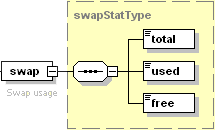The swap node describing virtual memory (swap) usage on the server is presented by complex type swapStatType (`plesk_server.xsd`). The node is structured as follows:• The total node is required. It returns the total amount of swap (in bytes) configured on the server. Data type: size (`common.xsd`).
• The used node is required. It returns the amount of swap (in bytes) being used by different processes on the server. Data type: size (`common.xsd`).
• The free node is required. It returns the amount of free swap (in bytes) available on the server. Data type: size (`common.xsd`).

The part of the response packet returning swap usage statistics may look as follows:

```<swap>
<total>419430400</total>
<used>327544832</used>
<free>91885568</free>
</swap>
```